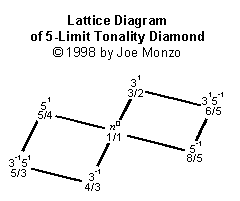## en français Deutsche Joe Monzo's Harmonic Lattice Diagrams Text and diagrams © 1998 by Joseph L. Monzo

The best way that I have discovered, to grasp as much harmonic information as possible in a just-intonation musical tuning system, is the use of lattice diagrams which portray pitches as points in multi-dimensional space connected by vectors.

In a just-intonation tuning system, each note is represented by a ratio which describes that note's relationship to another note, usually one that is used as a reference for the whole system. This reference tone has the ratio 1/1, also described as 1:1 or 1 to 1.

Any number can be factored into the series of prime numbers, each of which is a base which has an exponent that is either positive or negative, representing numbers >1 or <1, respectively, unless the exponent=0, which represents 1, the identity in multiplication.

Powers of 2 are all harmonically equivalent to this identity 1, thus, powers of 2 represent "octaves", and thus have no pronounced effect on the harmony, and may be eliminated, unless "octave" registration is specifically under consideration. Therefore the diagrams normally begin with prime-base 3.

My lattice diagrams treat each prime base as a unique dimension in space, with all the exponents radiating outward from the central 1/1, which is equivalent to all numbers to the 0th power, or n0.

Considered in 2-dimensional space, the vectors which connect each exponent travel in in a unique direction for each prime. This direction is determined by the angle which represents the cents value (within the "octave") of that prime to the 1st power, starting from 6 o'clock position.

Thus, 31, which is the ratio 3/2 and is 702 cents, has a vector radiating out from 1/1 at very close to 1 o'clock position (because 6 + 7 mod 12 = 1).

51, which is the ratio 5/4 and is 386 cents, has a vector radiating out at just less than 10 o'clock position (6 + 3.86 = 9.86), and so on.

The negative exponents simply radiate outwards from the opposite direction.

The vectors also vary in length and thickness according to the prime-bases they are connecting, with 3 the shortest and thickest.

Interestingly, even though 2-dimensional space was the milieu in which these measurements were made, they appear to they eye to form 3- or even more -dimensional structures, somewhat resembling crystals.

Harry Partch invented a structure he called the "Tonality Diamond". This shows all harmonic relationships of ratios with numbers under a certain "Odd Limit" (excluding "octave doubling", or multiplication by 2, which he used to keep each ratio within the same "octave", as in most usual source scales).

These "diamonds", which simply get larger and hold exponentially more ratios with each new further odd-limit in Partch's version, take on a completely different aspect when illustrated using my lattices. The process certainly appeals to my artistic sensibilities.

I illustrate here the 5-Limit, 7-Limit, 9-Limit, 11-Limit, and 13-Limit "Tonality Diamonds" as represented by my lattice diagrams.

The symmetry which Partch noted in his scales and Tonality Diamonds is readily apparent here, although the Tonality Diamonds in this representation are not perfectly symmetrical, as are some other systems I have devised.

Partch called this the Incipient Tonality Diamond, because a triad with the proportions 4:5:6 gives the maximal consonance for a chord with three distinct identities, thus this is the simplest system that can be constructed which gives any real feeling of tonality.

The "major" triad on my lattice looks like this (with odentities given):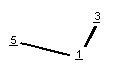and the "minor" triad looks like this (with udentities):Note that the 5-Limit Lattice or Diamond contains 3 each of the "major" and "minor" triads. Each n-Limit system always contains (n+1) chords of maximal consonance, each with (n+1)/2 identities, evenly divided into (n+1)/2 each of "major" and "minor".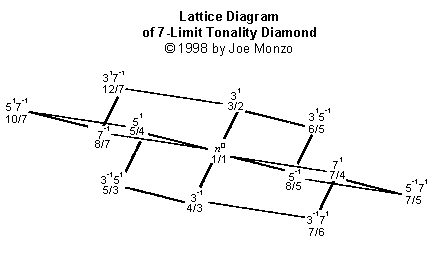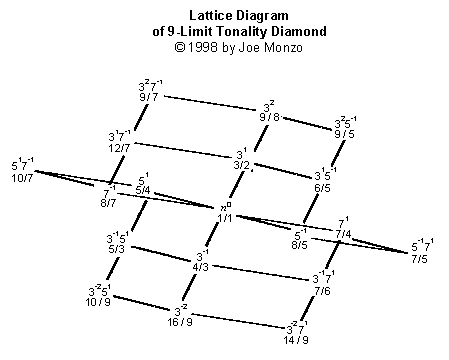Note that the 9-Limit Lattice does not have a new dimension -- it merely adds a layer, at either end of the powers of 3, onto the 7-Limit Lattice. This is because 9 is not a prime number, but rather a composite, that is, a number made up of other primes, in this case
3 x 3, or 32.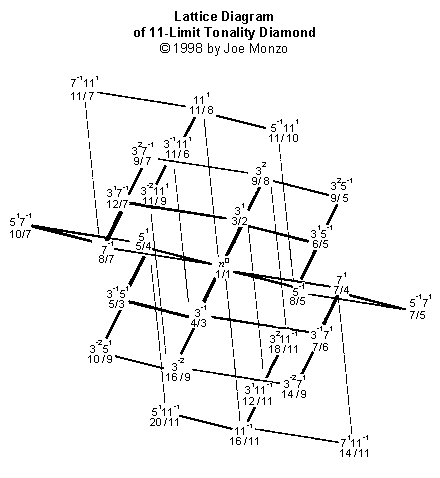Here's a version of the 11-Limit Lattice in full color: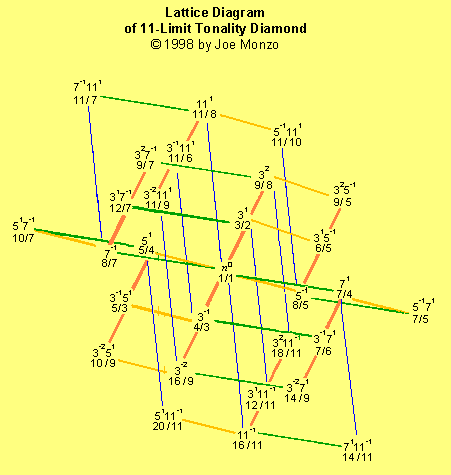Next is a shaded version of the 11-Limit Lattice which I like most of all: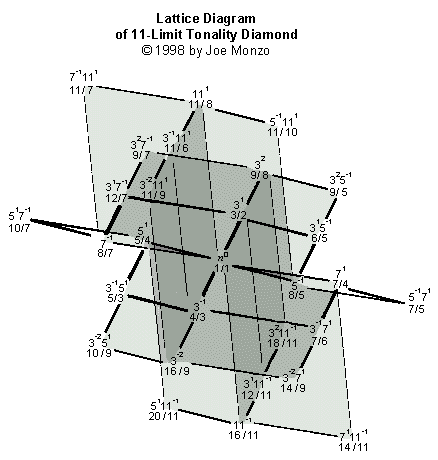And most complicated of this set, the 13-Limit Lattice: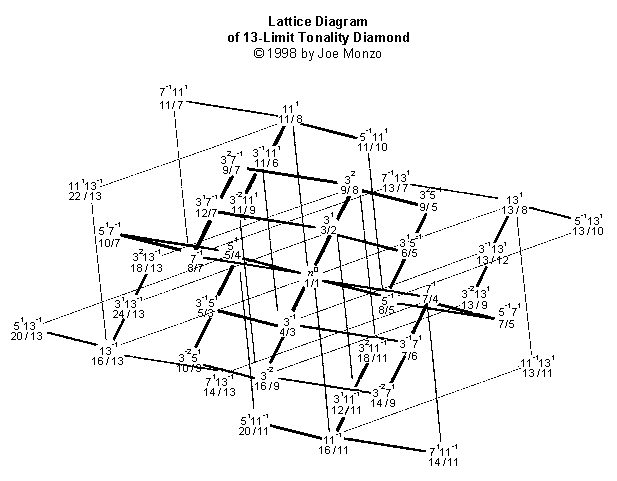The lattices form intricate crystal-like structures that show a wealth of harmonic information at a glance. If they are viewed in the order listed above, it can be seen that each smaller one is contained within the larger ones.

Below is a lattice using my usual conventions, except that the angle for each prime-axis is the "golden angle" of ~222.49 degrees, and the length of one step on each axis is log(prime). The idea was suggested by Paul Erlich (Yahoo tuning list message 22065 Thu May 3, 2001 2:38 pm):

The idea would be to mirror the way many plants and flowers grow. First, a single "bud" pops out of the center. After the first bud has grown a bit, the next "bud" pops out at the golden angle (222.49 degrees). Then the next bud pops out at the golden angle relative to that. And so on. Each bud would represent a different prime. This would ensure that, if you used a lot of different primes in your lattice, their lines would stay as far as possible from one another and near-overlaps would be avoided.

This is the basic mapping of primes on the lattice: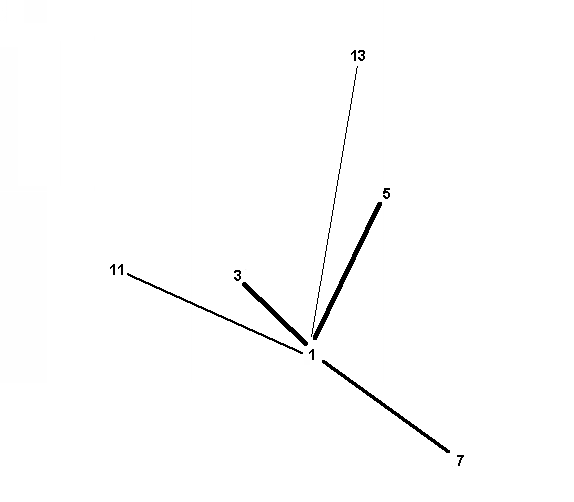And this, as one example, is the 11-limit tonality diamond: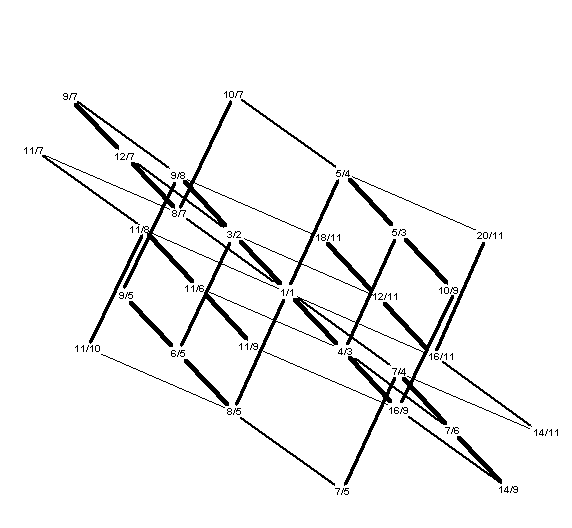Updated:

 For many more diagrams and explanations of historical tunings, see my book. If you don't understand my theory or the terms I've used, start here or try some definitions.

 I welcome feedback about this webpage: corrections, improvements, good links. Let me know if you don't understand something.return to my home pagereturn to the Sonic Arts home page

Thanks to Ming Sun Ho for the nice addition below.

The best and most recent version of Ming's applet is here, without the background.